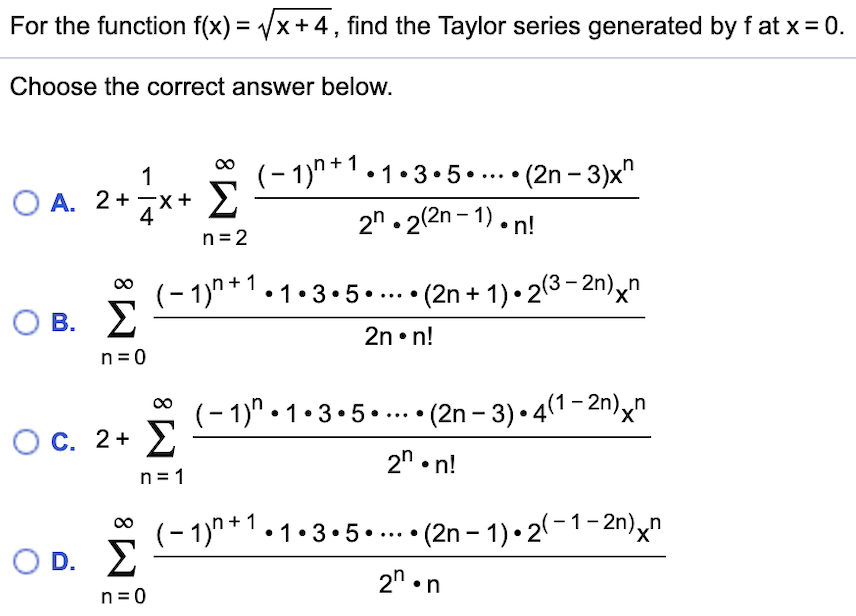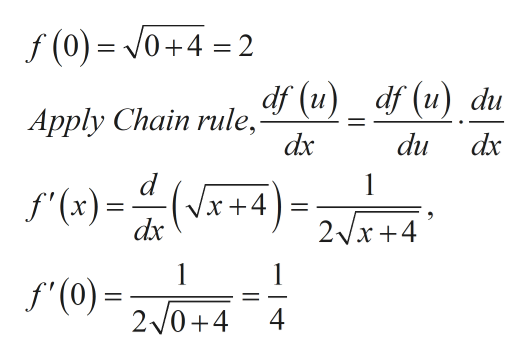# For the function f(x) = /x+ 4,find the Taylor series generated by f at x0.Choose the correct answer below.(-1)n+1.1.3.5. Σ(2n -3)x"1O A. 2+X2n.22n-1).n!n 2(-1)1-3.5..... (2n + 1)-23-2n)nΣ_O B.2n. n!n 0(-1)" 1-3.5..(2n -3) 41-2n)^"ΣX'O C. 2+2" n!n 1(-1)"1.3.5..... (2n -1)-2(-1-2n)xnΣ_X'D.2n nn 0

Question
6 views

Kindly helphelp_outlineImage TranscriptioncloseFor the function f(x) = /x+ 4,find the Taylor series generated by f at x 0. Choose the correct answer below. (-1)n+1.1.3.5 . Σ (2n -3)x" 1 O A. 2+ X 2n.22n-1).n! n 2 (-1)1-3.5..... (2n + 1)-23-2n)n Σ _ O B. 2n. n! n 0 (-1)" 1-3.5..(2n -3) 41-2n)^" Σ X' O C. 2+ 2" n! n 1 (-1)"1.3.5..... (2n -1)-2(-1-2n)xn Σ _ X' D. 2n n n 0 fullscreen
check_circle

Step 1

Refer to the question, we have to calculate the Taylor series for the provided function,

Step 2

Now use the taylors formula to  find the series which is,

Step 3

Then find the derivative of f(x) at x=0 and substit...help_outlineImage Transcriptionclosef (0) 0+4 =2 Apply Chain rule, (u) _ df (u) du du dx dx d x+4 dx 1 f'(x) = 2x+4 1 1 f'(0)20+4 4 fullscreen

### Want to see the full answer?

See Solution

#### Want to see this answer and more?

Solutions are written by subject experts who are available 24/7. Questions are typically answered within 1 hour.*

See Solution
*Response times may vary by subject and question.
Tagged in

### Calculus